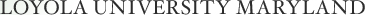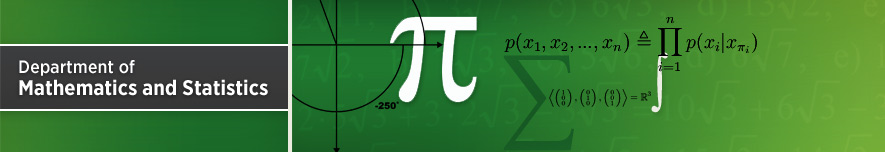## Dr. Mariah Hamel

### Room: 007 Knott Hall

Abstract:

Arithmetic combinatorics is a quickly growing field that can be described as the study of structural properties of sets. For example, Roth's theorem on three term arithmetic progressions states that any 'large' subset of the natural numbers contains a solution to the equation $$\displaystyle x+z=2y$$. This area has attracted the interest of those who study analysis, combinatorics, number theory and ergodic theory. In this talk we will describe several historical results and explain why the discrete Fourier transform is a useful tool in arithmetic combinatorics.

Back to Current Seminars page